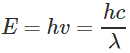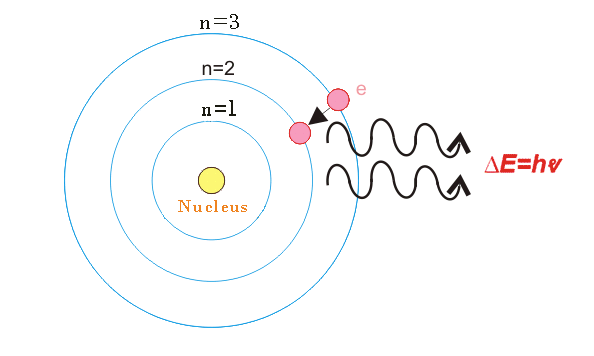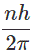# Bohrs Atomic Model

It is introduced by Danish Physicist Niels Bohr in the year of 1913. According to this model the atom consists of small nucleus at the center and electrons which rotates in circular orbits surrounding the nucleus – similar to solar system. But here, the force of attraction is provided by electrostatic forces rather than gravitational forces. The nucleus is positively charged and electrons are negatively charged. Further Niels Bohr illustrated that the positively charged nucleus consists of protons and neutrons. The protons are positively charged and neutrons does not have any charges. Niels Bohr introduced the quantum theory to overcome the drawbacks of Rutherford’s atomic model. According to this theory –

1. Electrons revolves around the nucleus in certain orbits. Each orbit is having certain energy level. These orbits are called stationary orbits. The orbit near to nucleus is having low energy level and the outer orbit is having higher energy level.An electron can revolves in certain energy level without losing any energy. On addition of energy to the atom, the electron jumps to orbit of higher energy level.
On the other hand, when electron jumps from the orbit of higher energy level to the orbit of lower energy level, the electron release the energy in small packets. These small packets are called the quanta or photons. The energy of photon is given by,2. Where,
‘h’ is the plank’s constant,
‘υ’ is the frequency of light (in Hz),
‘c’ is the speed of light (in m/sec),
‘λ’ is the wave length of light emitted (in meter).3. The centripetal force due to electrostatic attraction between positively charged nucleus and negatively charges electron is equal to the centrifugal force of electron moving in circular orbits.
4. The angular momentum of electron moving in circular orbits is the integral multiple ofWhere, n is an integer called quantum number.
5. The radius of the orbit is proportional to n2 and the velocity of electron is inversely proportional to n. These assumptions led to results which have been found correct of being tested.

This model is also having some deficiency which are listed below-

1. It is applied to one electron atom i.e. hydrogen atom. It cannot be easily extended to explain the more complicated atoms.
2. It does not give any rule or restriction regarding the transition of an electron from one orbit to another orbit.
3. It introduced only one quantum number n. Whereas, experimental evidence concerning fine structure of spectral line suggests the more additional quantum numbers.
4. The quantitative explanation of chemical bonding cannot be explained by the Bohr’s atomic model.

Want To Learn Faster? 🎓
Get electrical articles delivered to your inbox every week.
No credit card required—it’s 100% free.